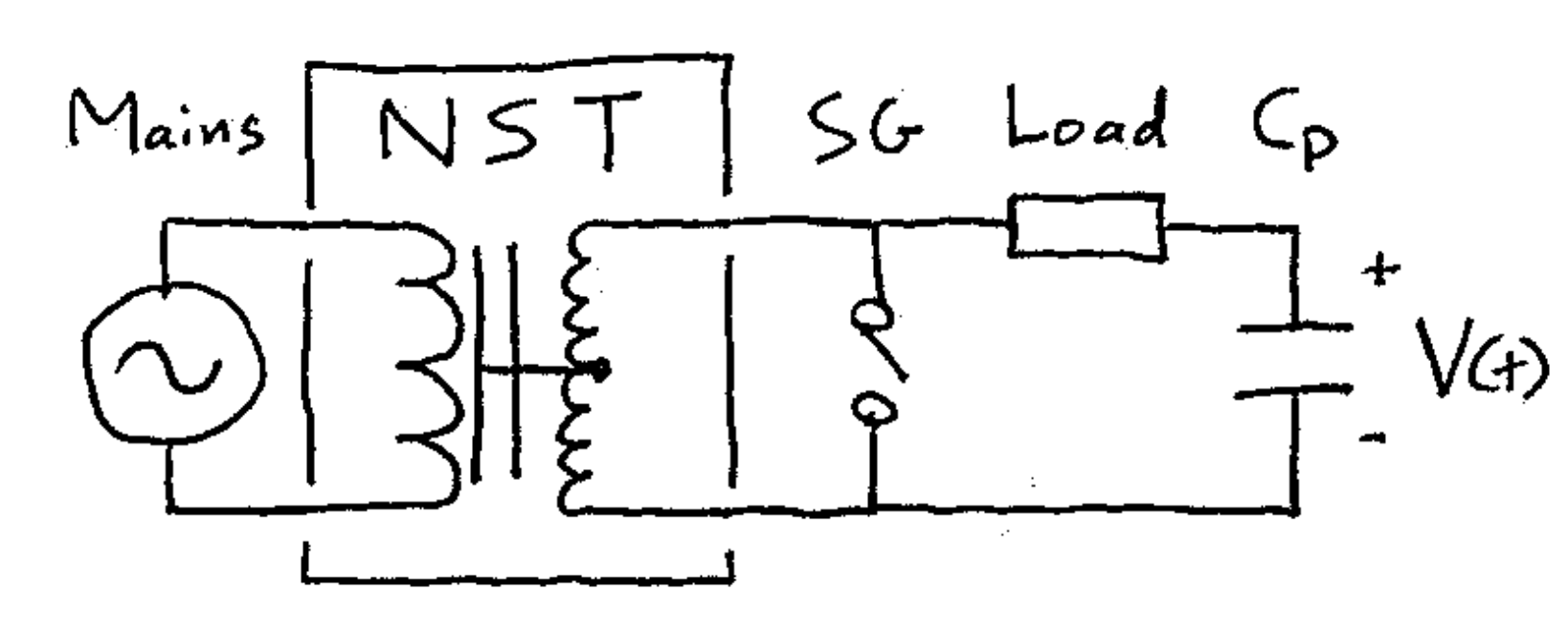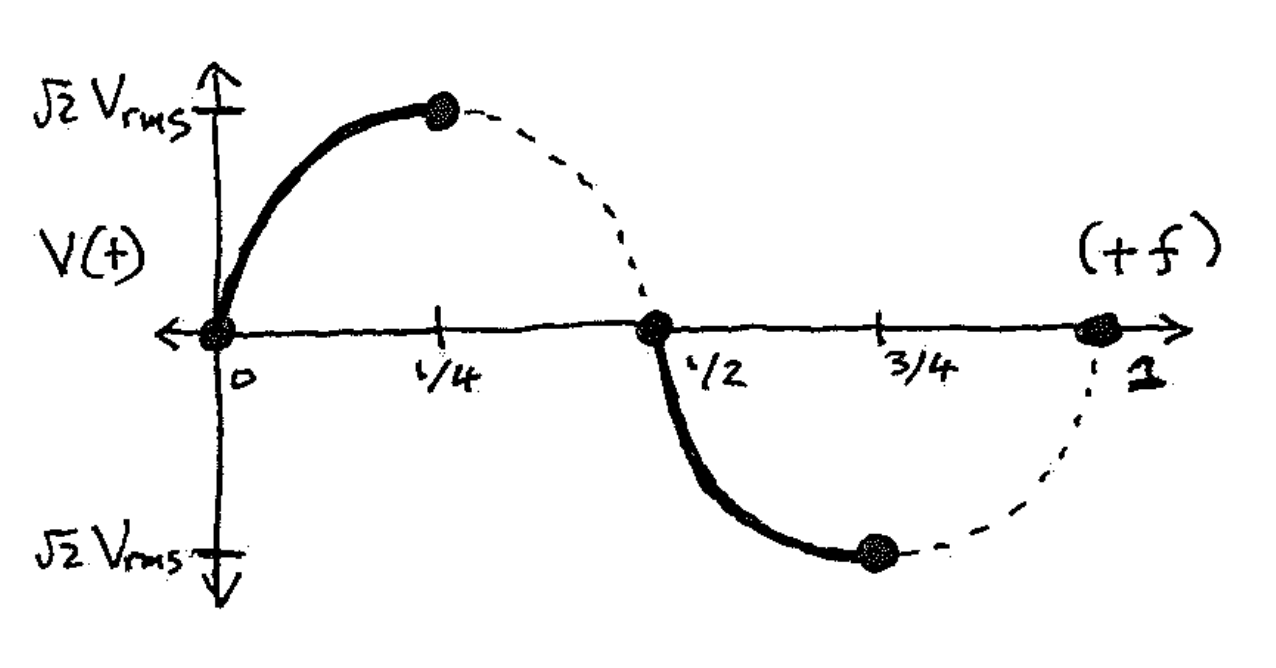Tech Notes
##### Deriving the LTR primary capacitance formula for NSTs
###### TLDR: Derivation of a common formula used with Tesla coils.

While working on a project that used a neon sign transformer (NST) to repetitively charge and discharge a bank of capacitors, I needed to determine the maximum capacitance $$C_\text{max}$$ the bank could have without reducing the charging voltage. Approximating the NST as an ideal power supply (it isn't ...) led to a really simple formula for $$C_\text{max}$$. After doing this, I remembered that there is a formula for a capacitance $$C_\text{LTR}$$ commonly used to design Tesla coils that should apply, even though that wasn't what I was building. Surprisingly, the two formulas are nearly identical. I realized I didn't know where the Tesla coil formula came from, so I thought I'd dig in more. Here's what I found.

First, let's derive $$C_\text{max}$$. Consider an AC power supply with frequency $$f$$ (e.g., 60 Hz), rated output power $$P = V_\text{rms} I_\text{rms}$$ and output impedance $$Z = V_\text{rms}/I_\text{rms}$$, where $$V_\text{rms}$$ and $$I_\text{rms}$$ are the maximum root-mean-square output voltage and current used to specify NSTs. Then $$C_\text{max}$$ corresponds to the capacitance that charges from zero voltage up to $$\sqrt{2}\, V_\text{rms}$$ during a quarter cycle, or $$1/(4f)$$ seconds, at the power $$P$$. Energy conservation requires $$(1/2) C_\text{max} (\sqrt{2} \, V_\text{rms})^2 = P / (240~\text{Hz}),$$ giving $$C_\text{max} = 1/(4 f Z).$$ For 60 Hz AC, $$C_\text{max} = 1/(Z \times 240~\text{Hz}).$$ This result assumes the supply is a sine-wave voltage source (see Figure 1) and that its current does what's needed so the time-averaged power over a quarter cycle is $$P$$.###### Figure 1: (Left) Example circuit for NST charging of the primary capacitor $$C_p$$ in a Tesla coil. Acronyms: NST: neon sign transformer; SG: spark gap. (Right) Solid curves are the voltage across $$C_p$$ during charging, assuming the NST acts as an ideal sine-wave voltage source and the "Load" is negligible. In a Tesla coil, this voltage would not follow the dashed curves because of the spark gap firing.

Next, here's background on the Tesla coil formula. While designing a spark-gap Tesla coil, one step is to choose the primary tank circuit's capacitance $$C_p$$, which controls the amount of energy in each operational cycle (the "bang size"). For simple static spark gaps often used with NSTs, the recommended choice for $$C_p$$ is $$C_\text{LTR} = 1.6 / (2 \pi f Z),$$ which is called the static-gap "larger-than-resonant" (LTR) capacitance . The rounded factor of "1.6" seems to be the original choice, but sometimes is replaced with another value between 1.5 and 2.0. For 60 Hz AC, $$C_\text{LTR} \approx 1 / (Z \times 236~\text{Hz})$$ which is almost identical to $$C_\text{max}$$ above.

The similarity between $$C_\text{LTR}$$ and $$C_\text{max}$$ is not accidental. It took some digging, but you can read about the origins of $$C_\text{LTR}$$ in [2-3]. In short, $$C_\text{LTR}$$ first came from computer simulations of NST charging in Tesla coils . These simulations seem to have treated the NST as a simple transformer (with nearly unity coupling) that behaves like an ideal power supply until the spark gap fires. $$C_\text{LTR}$$ then came from finding the capacitances that gave 120 breaks per second (BPS) for each NST (i.e., charging in a quarter cycle). Moreover, a little later, $$C_\text{LTR}$$ was justified analytically with essentially the same derivation as that given for $$C_\text{max}$$ above . So, the two formulas should be identical — neat!

Since it's so hard to find the derivation of $$C_\text{LTR}$$ , and it doesn't seem to be well known, I thought it'd be worth sharing this note. Last but not least, before you use these formulas, remember that NSTs are not ideal power supplies, so the optimal primary capacitance $$C_p$$ will come from trial and error (thus the varying factor of "1.6") . $$C_\text{LTR}$$ and $$C_\text{max}$$ are just design guides to help get you started.

References

(January 2018; updated April 2018)